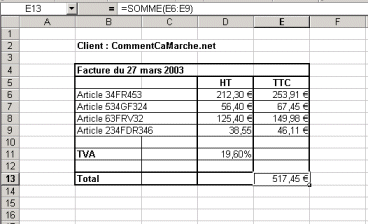# Introduction to Spreadsheet Formulas: What Is It and How to Use

Excel spreadsheets are one of the most useful tools to create documents with calculations and data and organize the accounting or VAT tax, among other tasks. Luckily, while working on Excel you can use various formulas to speed up and facilitate the process. In this article, we will show you what it is and how to use formulas.The main use of a spreadsheet is to automate calculations, which means using cells to perform operations based on values in other cells. The spreadsheet recalculates all the values each time a change is made to the value of a cell. A formula is used to represent an expression, which, one entered into the cell, allows the spreadsheet to
perform automatic calculations based on values in other cells and to display the result. Formulas may also contain references to other cells, expressions, or functions.

## How to Enter a Formula

Entering a formula is done by selecting a cell and by using the formula bar.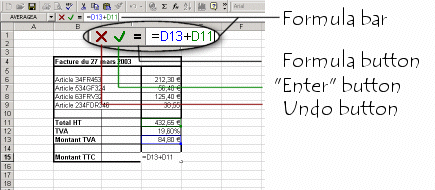To enter a formula, start by entering the equal sign (=) and then enter the cell references of the cells to be used and the operators and/or functions.

It is also possible to click on the button Formula on the formula bar. The example below demonstrates the use of a formula in cell C15 to calculate the sum of cells D11 and D13.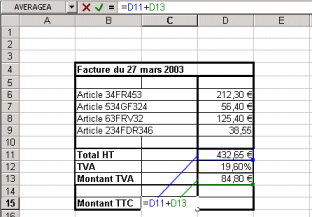When the Enter key is pushed, the spreadsheet calculates the value of cell C15 and displays the result: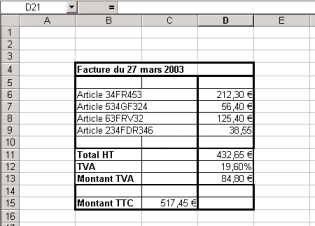## Practical Example

In the example below, the use of formulas to automatically calculate the price with tax included (Tax included) of a list of articles for which the "Before Tax" (BT) price is given, and then to calculate the total price of these articles is shown.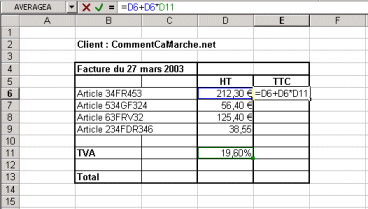To obtain the Tax Included price for article 34FR453, add the value of the tax to the pre-tax value, meaning the pre-tax value multiplied by the VAT (19.6% in this case):

`Amount_TI = Amount_BT + Amount_BT*VAT`

In the above example,

• the TI amount is located in E6,
• the BT amount is located in D6,
• the VAT amount is located in D11,

which gives the following formula:

`= D6 + D6*D11`

To calculate the TI amount for article 34FR453, first select the cell corresponding to this calculation (E6) and then start entering the formula (either by starting with the equal sign or by clicking the formula button).

The spreadsheet is therefore in the formula entering mode, and:

• either enter the formula directly with the keyboard
• or build the formula by selecting the cells one at a time

In the second example, clicking on cell D6 will automatically add its name to the formula bar. Another click on this cell will display D6+D6 because addition is the default operation in the spreadsheet. Push the * button to choose multiplication, and then select cell D11.

To finish entering the formula, push the Enter button on the keyboard or use the Enter button on the formula bar. The spreadsheet will display the result of the calculation in cell E6: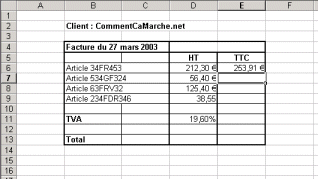## How to Copy Formulas

Imagine that we want to copy this formula and use it for the other articles. Select the formula in (cell E6), copy it, and select the block of cells from E7 to E9 (written E7:E9) and paste the previously copied formula. Here is the result: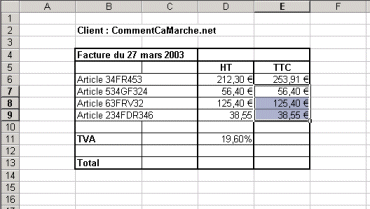Note that the copied formula does not produce the correct amounts: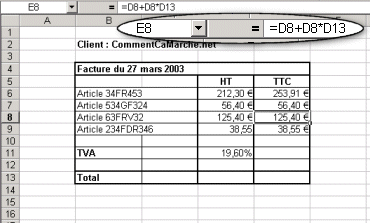In effect, when clicking on one of these cells, it can be noticed that the spreadsheet references the correct price before tax, but that the reference to the cell with the VAT value is

This is because the previously entered references were relative references.
The spreadsheet, therefore, performs an automatic offset on cell references when copying the formula. Thus, to avoid the spreadsheet offsetting the cell with the VAT value, use an absolute reference (\$D\$11). Let's redo the formula: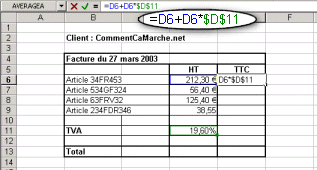The spreadsheet now gives a coherent result: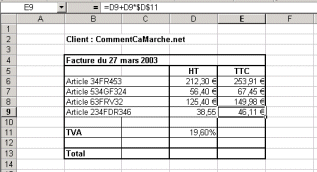## How to Use Functions in Formulas

Functions may also be used in formulas, thus allowing advanced calculations. Most
spreadsheets offer a large number of integrated functions (Excel has more than 400).

Let's redo the above example. To automatically calculate the pre-tax total and the TI total of orders, use the Sum() function. To do this, select the cell in which to display the sum and enter the formula (starting with the = sign) using the Sum() function. There are many ways of entering the Sum() function:

• by manually entering it in the formula bar
• by using the function button that allows the function to be chosen from a list: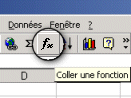• by using the sum button directly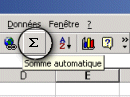Whatever method chosen, the two arguments of the Sum() function must be specified, which means specifying the cells for which the sum is to be calculated. There are two further possibilities for the user:

• enter the references of the selected cells on the keyboard
• select the cells or the blocks of cells to be used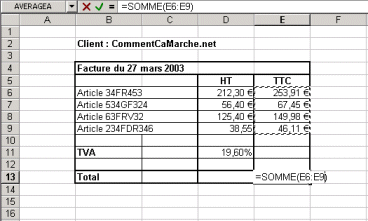Here is the result calculated by the spreadsheet: